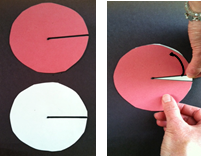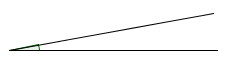# Shapes and Angles

## Objective

Understand that an angle that turns through ${{1\over360}}$ of a circle is called a “one-degree angle” and can be used to measure angles. Use a makeshift protractor to measure benchmark angles.

## Common Core Standards

### Core Standards

?

• 4.MD.C.5 — Recognize angles as geometric shapes that are formed wherever two rays share a common endpoint, and understand concepts of angle measurement:

• 4.MD.C.6 — Measure angles in whole-number degrees using a protractor. Sketch angles of specified measure.

## Criteria for Success

?

1. Understand that the unit of measure for angles is a degree, which is the measure of $\frac{1}{360}$ of a turn of a circle, and that all angles are measured with iterations of this unit.
2. Understand that the degree measure of angles can be measured using a tool called a protractor.
3. Measure benchmark angles with a paper protractor made up of 30° or 45° angles.
4. Start to internalize benchmark angles, both what they visually look like and what their degree measure is (right angle, 30° angle, etc.) (MP.2).

## Tips for Teachers

?

• Wedges were constructed in Lesson 5 Anchor Task #2 from blank paper circles.
• Teachers will need two paper circles for this lesson. For the paper circles, you can use parchment paper baking circles, as in Lesson 5. You might prefer two paper circles of different colors to help distinguish angles more easily. This different-colored paper circle can be made from tracing your existing paper circle onto a colored sheet of paper and cutting along the tracing. Use them to construct a paper protractor as follows before the lesson:
1. Label and cut a radius into each paper circle (shown as red and white circles at right).2. Stack the red circle on top of the white circle with the radii aligned and parallel to the desk’s edge.
3. Pinch the corner of the white circle directly below the slit.
4. To illustrate an angle, turn the segment given by the edge of the white region counterclockwise.

(Reference: Engage NY, Grade 4 Mathematics, Module 4, Topic B, Lesson 5)

• To help students build their visual models of benchmark fractions, you can use the applet Estimating Angles by NRICH. You can play as a whole class when there are a few minutes to spare, have individual students play online, or refer parents to the website.

### Lesson Materials

• Wedge (1 per student) — These were constructed in Lesson 5 using paper circles
• Paper circle (2 per student)
• Circular protractor (1 per student)

#### Fishtank Plus

• Problem Set
• Student Handout Editor
• Vocabulary Package

?

### Problem 1

Measure the following angle with your wedge.#### References

EngageNY Mathematics Grade 4 Mathematics > Module 4 > Topic B > Lesson 5Concept Development Problem 2

Grade 4 Mathematics > Module 4 > Topic B > Lesson 5 of the New York State Common Core Mathematics Curriculum from EngageNY and Great Minds. © 2015 Great Minds. Licensed by EngageNY of the New York State Education Department under the CC BY-NC-SA 3.0 US license. Accessed Dec. 2, 2016, 5:15 p.m..

Modified by Fishtank Learning, Inc.

### Problem 2

Using the circular protractor, determine how many degrees a quarter turn, or right angle, measures. Then do the same for a half turn, or straight angle, a three-quarter turn, and a complete circle or rotation.

#### References

EngageNY Mathematics Grade 4 Mathematics > Module 4 > Topic B > Lesson 5Concept Development Problem 2

Grade 4 Mathematics > Module 4 > Topic B > Lesson 5 of the New York State Common Core Mathematics Curriculum from EngageNY and Great Minds. © 2015 Great Minds. Licensed by EngageNY of the New York State Education Department under the CC BY-NC-SA 3.0 US license. Accessed Dec. 2, 2016, 5:15 p.m..

Modified by Fishtank Learning, Inc.

### Problem 3

Determine how many degrees the wedge created in Lesson 5 measures, and use that to create a makeshift protractor to measure benchmark angles.

Then, use a similar process to create a protractor that measures in increments of 45°.

#### References

EngageNY Mathematics Grade 4 Mathematics > Module 4 > Topic B > Lesson 5Concept Development Problem 3

Grade 4 Mathematics > Module 4 > Topic B > Lesson 5 of the New York State Common Core Mathematics Curriculum from EngageNY and Great Minds. © 2015 Great Minds. Licensed by EngageNY of the New York State Education Department under the CC BY-NC-SA 3.0 US license. Accessed Dec. 2, 2016, 5:15 p.m..

Modified by Fishtank Learning, Inc.

## Problem Set & Homework

### Discussion of Problem Set

• A full turn is 360°. What could you do to find the degree measure of an angle that takes 10 turns to make a whole turn?
• Shade in the region of a 45° angle on your eighths circle. What fraction of the whole turn is that? Do the same for your 30° angle.
• What if you shaded in a region defined by a 120° angle on your twelfths circle? What fraction of the whole circle is that?
• Use your protractor to explain to your partner what a degree is.
• Use your protractor to trace some benchmark angles.
• If you didn’t have a protractor, how could you create one?

?

### Problem 1

What fraction of a complete turn through a circle is 1°?

### Problem 2

What is the measure, in degrees, of a right angle?

### Problem 3

List the measurements, in degrees, of three benchmark angles.